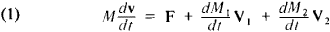# Mechanics of Bodies of Variable Mass

The following article is from The Great Soviet Encyclopedia (1979). It might be outdated or ideologically biased.

## Mechanics of Bodies of Variable Mass

a branch of theoretical mechanics that deals with the motions of material bodies whose mass varies during the motion. The founders of the mechanics of bodies of variable mass were I. V. Meshcherskii and K. E. Tsiolkovskii. Problems in the mechanics of bodies of variable mass have arisen in the development of aeronautical and rocket engineering and theoretical mechanics.

A change in the mass of a body (point) during motion of the body may be due to the separating out (ejection) or joining up (capture) of particles. Processes of both capture and ejection of particles take place simultaneously during the flight of modern jet-propelled aircraft having air-breathing jet engines. Such aircraft gain mass because of the air particles sucked into the engine and lose mass because of the ejection of particles—the products of fuel combustion. The principal vector differential equation of motion for a point of variable mass for the case of both capture and ejection of particles (first derived in 1904 by Meshcherskii) has the formwhere V1 is the relative velocity of the ejected particles, ǀdM1/dtǀ is the mass flow out from the moving point per second, V2 is the relative velocity of the particles captured, and ǀdM2/dtǀ is the mass flow into the point per second. The product (dM1/dt) V1 = Φ1 is the reaction thrust, and (dM2/dt) V2 = Φ2 is the braking force due to the capturing of particles. For modern rockets, the equation of motion is obtained from equation (1) by putting Φ2 = 0; this equation of motion was derived by Meshcherskii in 1897.

Two classes of problems are considered in the mechanics of bodies of variable mass: the determination of the trajectory of the center of mass and the determination of the motion of the body of variable mass about the center of mass. In a number of cases, the trajectory characteristics of the motion of the center of mass can be found from the dynamical equations of the point of variable mass. The study of the motion of a body of variable mass about the center of mass is important for investigating the dynamic stability of real objects (rockets and aircraft) and their controllability and maneuverability. Problems in the mechanics of bodies of variable mass also include the search for optimal modes of motion, that is, the determination of the form of the variation of mass of a body or point for which the kinematic or dynamic characteristics of the motion become optimal. The most effective method of solving such problems is the calculus of variations.

An important problem in the mechanics of bodies of variable mass that have a rigid shell is the study of the motion of these bodies when certain supplementary conditions are imposed on the velocity of the center of mass. Such problems arise, for example, in the study of the motion of remote-controlled rockets and unpiloted aircraft that are guided on target automatically or by radio commands from the ground. Many studies on the mechanics of bodies of variable mass pertain to the study of the motion of celestial bodies. If it is assumed that the increase in the mass of a celestial body results from the capture of cosmic dust, one reaches the supplementary condition that the relative velocity of the incoming particles is zero.

### REFERENCES

Tsiolkovskii, K. E. Sobr. soch., vol. 2. Moscow, 1954.
Meshcherskii, I. V. Raboty po mekhanike telperemennoi massy, 2nd ed. Moscow, 1952.
Kosmodem’ianskii, A. A. Mekhanika tel peremennoi massy, part 1. [Moscow] 1947.
Kosmodem’ianskii, A. A. Kurs teoreticheskoi mekhaniki, 3rd ed., part 2. Moscow, 1966.
Miele, A. Mekhanika poleta (teoriia traektorii poleta). Moscow, 1965. (Translated from English.)

A. A. KOSMODEM’IANSKII